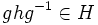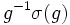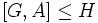# Normal and self-centralizing implies coprime automorphism-faithful

This article gives the statement and possibly, proof, of an implication relation between two subgroup properties. That is, it states that every subgroup satisfying the first subgroup property (i.e., self-centralizing normal subgroup) must also satisfy the second subgroup property (i.e., coprime automorphism-faithful normal subgroup)
View all subgroup property implications | View all subgroup property non-implications
Get more facts about self-centralizing normal subgroup|Get more facts about coprime automorphism-faithful normal subgroup
This article states and (possibly) proves a fact about a finite group and a Coprime automorphism group (?): a subgroup of the automorphism group whose order is relatively prime to the order of the group itself.
View other such facts

## Statement

### Verbal statement

Any normal Self-centralizing subgroup (?) of a finite group is coprime automorphism-faithful.

### Statement with symbols

Suppose$G$ is a finite group and$H$ is a normal subgroup of$G$ such that$C_G(H) \le H$ (or equivalently,$C_G(H) = Z(H)$). Then, if$\sigma$ is a non-identity automorphism of$G$ whose order is relatively prime to the order of$G$, and if$\sigma(H) = H$, then the restriction of$\sigma$ to$H$ is a non-identity automorphism of$H$.

## Proof

### Hands-on proof

Given: A finite group$G$, a normal subgroup$H$ such that$C_G(H) \le H$. An automorphism$\sigma$ of$G$ that acts as identity on$H$, and such that the order of$\sigma$ is relatively prime to the order of$G$

To prove:$\sigma$ is the identity automorphism

Proof: Note that for$g \in G, h \in H$, we have, by normality, that$ghg^{-1} \in H$. Thus, since$\sigma$ acts as the identity on$H$, we get:$\sigma(ghg^{-1}) = ghg^{-1}$.

Expand the left side and use that$\sigma(h) = h$ to obtain:$\sigma(g)h\sigma(g)^{-1} = ghg^{-1}$.

Rearranging this yields that$g^{-1}\sigma(g)$ commutes with$h$. Since this holds true for every$h \in H$, we obtain that$g^{-1}\sigma(g) \in C_G(H)$. Since$C_G(H) \le H$ by assumption, we obtain that$g^{-1}\sigma(g) \in H$, and thus,$g$ and$\sigma(g)$ are in the same coset of$H$ in$G$. Thus,$\sigma$ stabilizes the subnormal series:$\{ e \} \triangleleft H \triangleleft G$.

Thus, by fact (2),$\sigma$ must be the identity automorphism.

### Proof using three subgroup lemma

Note that this proof superficially appears different from the preceding proof, but the part of the proof using the three subgroup lemma is precisely equivalent to the initial part of the proof above upto the point where we deduce that$g^{-1}\sigma(g) \in H$. Thinking of the proof in terms of the three subgroup lemma, rather than in terms of step-by-step manipulations as outlined above, is more useful in some situations.

Given: A finite group$G$, a normal subgroup$H$ such that$C_G(H) \le H$. An automorphism$\sigma$ of$G$ that acts as identity on$H$, and such that the order of$\sigma$ is relatively prime to the order of$G$

To prove:$\sigma$ is the identity automorphism

Proof: Suppose$A$ is the subgroup generated by$\sigma$ in$\operatorname{Aut}(G)$, and let's assume we're working in$G \rtimes A$. By assumption,$[H,A]$ is trivial. Since$H$ is normal in$G$, we have$[G,H] \le H$. Thus, we have:

•$[[G,H],A] \le [H.A]$, which is trivial, so$[[G,H],A]$ is trivial
•$[[H,A],G]$ is trivial, because$[H,A]$ is trivial

So, by the three subgroup lemma,$[[G,A],H]$ is trivial. Since$[G,A] \le G$, we see that$[G,A] \le C_G(H)$, so by the assumption of self-centralizing, we obtain that$[G,A] \le H$. Thus,$A$ stabilizes the subnormal series:$\{ e \} \triangleleft H \triangleleft G$

Now, using the fact that the stability group of the subnormal series of a finite group has no other prime factors, we conclude that$A$ must be the trivial group, so$\sigma$ is the identity automorphism.